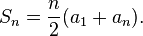# Are you better at math or code?

“Choose a number from 1 to 10” – they usually choose 5, I’m not sure why.

This how an interview question I’ve used to ask begins…

### The question

Suppose you’re writing a client that receive a message with a number N (usually 5). Next you’ll receive N messages each contains a different number from 1 to N

1 2 3 4 5

Simple. At this point the interviewee start looking for a catch, but being a kind soul that I am I reassure him that it’s yet to come…
Now to make things interesting the ordering of the messages (i.e. numbers) is not defined – in face you can receive the numbers in any order.

For example I could receive 2 3 4 1 5 or 3 5 1 3 4

Still here?
And finally we know for certain that one of the numbers will not be sent while another will be sent twice

For example: 2 3 1 5 1

That’s it. So now design an algorithm that will return two pieces of data – which number was not sent and which was sent twice. in the last example the output should be 4 and 1.
That a few minutes to think on how to solve this problem.

The reason I used to ask this question is that you can learn quite a lot by the way that this question is answered. The trick is that it’s actually two separate questions:

1. Which number appeared twice
2. Which number was not sent

As software developers we’re expected to have some math skills and (hopefully) can code.
I’ve noticed that developers with strong math skill usually answer one first while developers that feel more comfortable with code choose to answer the other. Most answered both but it’s the way that counts.
Note: Just to make things clear – I believe that good developers are good in both fields but usually are better at one than the other.

### Which number appeared twice

To find out which number appears twice you’ll need an array of size N (which we know) of Boolean or integers and each time a number arrive just go to that number’s index (or index – 1 because the array starts with ‘0’) if it’s marked false (or 0) just change that cell value to true (or 1) but if it was already previously changed you know that this is the number that appears twice – simple.
I found the developers that are stronger in code find this solution faster then the other.

### Which number was not sent

Most math centric developers quickly look at the input and see that it’s an arithmetic progression – a sequence of consecutive numbers and knowing that they also know that the sum of these numbers can easily be calculated:The sum on our example (again 1 to 5) is (1 + 5) X 5 / 2 = 15.
Now we have a sum without the number that appeared twice – no problem. In math there is a trick of assuming that we’ve solved the first question (which number appeared twice) all we need to do is add it to the sum so in our example 1 is added to result in 16.
After that all you need to do is sum the numbers as they arrive and in the end subtract the new sum from the expected and get the number that was not sent:
16 – (2 + 3 + 1 + 5 + 1) = 16 – 12 = 4.

### Conclusion

I don’t think that being better in one over the other makes a better programmer the only reason I’ve asked this question is to find if the candidate had basic skills at both and which of these he’s better – a way to understand who am I talking to.
I hope that you took the time to solve the question before reading the solution so now you know which field you feel more comfortable in.

Happy coding…

## 3 thoughts on “Are you better at math or code?”

1.Ken Egozi says:

oh I rock – solved it 🙂

loved this question – when I'll get back to interviewing I might use a similar one as well.

2.Guest says:

Does the developer solution not find the answer to both questions. If you're setting each of the elements in the array, then one will be left at 0. Doesn't this mean the one that is left at 0 is the one that didn't get sent? That is assuming you know N.

3.Ken Egozi says:

you'd need to re-scan the N length array in O(N) while keeping score in the input phase will give you the answer in O(1)

This site uses Akismet to reduce spam. Learn how your comment data is processed.